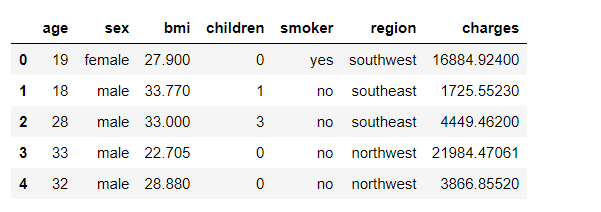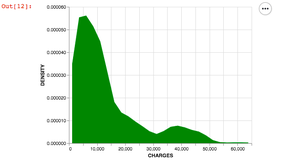# How To Make Density Plot in Python with Altair?

• Last Updated : 17 May, 2021

Density plots are a variation of Histograms that are used to observe the distribution of a variable in data set over a continuous interval or a time period. The peaks of a Density Plot indicate where values are concentrated over an interval.

Compared to Histograms, Density Plots are better at determining the distribution shape because they’re not affected by the number of bins.

## Density Plot in Python using Altair

We can make a density plot in python using the libraries Pandas and Altair.

• Altair-It is a statistical visualization library based on Vega and Vega-lite.
• Pandas-It is an open-source data analysis and manipulation tool in Python.

Note: We will be using the ‘insurance.csv’ dataset which can be downloaded from Google Drive.

First, let’s import these libraries using-

## Python3

 `import` `pandas as p  ``# loading pandas library``import` `altair as a  ``# loading altair library`

Next, we load the data set on which we need to use the density plot.

## Python3

 `data_set ``=` `'insurance.csv'`  `# dataset name``d ``=` `p.read_csv(data_set)  ``# reading the datasaet``d.head()  ``# printing the first 5 data entries`

Output:As you can see, there are seven columns in the dataset. We shall use “charges” to make a density plot. To do, so we must first transform our data into density. This is done by using the transform_density() function. The parameters are the variable of interest and a name to indicate the transformed variable which is written as “as_=[‘Charges’, ‘density’]”. Bringing it together-

## Python3

 `# loading a single column into``# the data frame object``d ``=` `d[[``"charges"``]]` `a.Chart(d).transform_density(``'charges'``, as_``=``[``'CHARGES'``, ``'DENSITY'``],``                             ``).mark_area(color``=``'green'``).encode(``    ``x``=``"CHARGES:Q"``,``    ``y``=``'DENSITY:Q'``,` `)`

Output:Output

Complete Script: Here is the code with all the steps at one place-

## Python3

 `import` `pandas as p  ``# loading pandas library``import` `altair as a  ``# loading altair library` `# download dataset from https://drive.google.com/drive/folders/1Dddv1l9hpEPVWh_uuK9Iv1A1xUNy55v7?usp=sharing``# OR replace name with your own dataset.``data_set ``=` `'insurance.csv'``d ``=` `p.read_csv(data_set)``d ``=` `d[[``"charges"``]]` `a.Chart(d).transform_density(``'charges'``, as_``=``[``'CHARGES'``, ``'DENSITY'``],``                             ``).mark_area(color``=``'green'``).encode(``    ``x``=``"CHARGES:Q"``,``    ``y``=``'DENSITY:Q'``,``)`

Output:Output

My Personal Notes arrow_drop_up